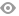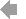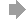﻿ Page 6 - REVISTA MATINF Nr. 5
Basic HTML VersionView Full Version
Page 6 - REVISTA MATINF Nr. 5
P. 6

``````6                                                                     L. Giugiuc, A.D. Pˆırvuceanu

Z  x
2     f(t)dt
For an arbitrary x ∈ (a, b), let k x :=   a         .
(x − a)(x − b)
From Cauchy’s Mean Value Theorem on the intervals [a, x] and [x, b] we get that ∃u x ∈ (a, x)
and v x ∈ (x, b) such that :

1
F(x)(2u x − a − b) = (x − a)(x − b)f(u x ) ⇐⇒ f(u x ) = (2u x − a − b)k x
2
1
F(x)(2v x − a − b) = (x − a)(x − b)f(v x ) ⇐⇒ f(v x ) = (2v x − a − b)k x
2

From Lagrange’s Mean Value Theorem on [u x , v x ] we get that ∃c x ∈ (u x , v x ) ⊂ (a, b) such
that
f(v x ) − f(u x )                       k x  2v x−a−b  −  2u x−a−b
0
0
= f (c x ) ⇐⇒ f (c x ) =        2         2     = k x ,
v x − u x                                  v x − u x
which is the desired conclusion.
References

 Gazeta Matematic˘a, No. 11/2019.
 Gazeta Matematic˘a, No. 5/2020.``````1   2   3   4   5   6   7   8   9   10   11